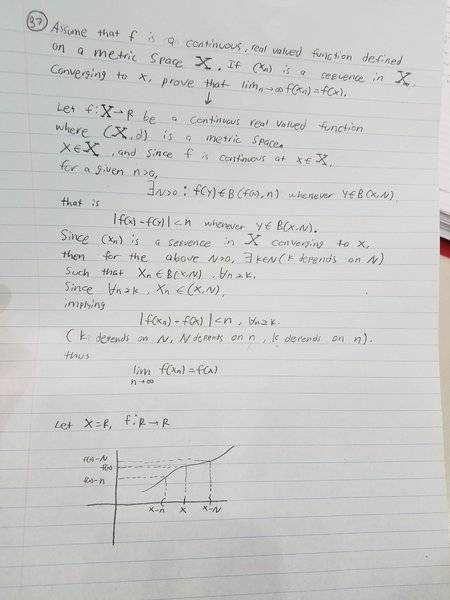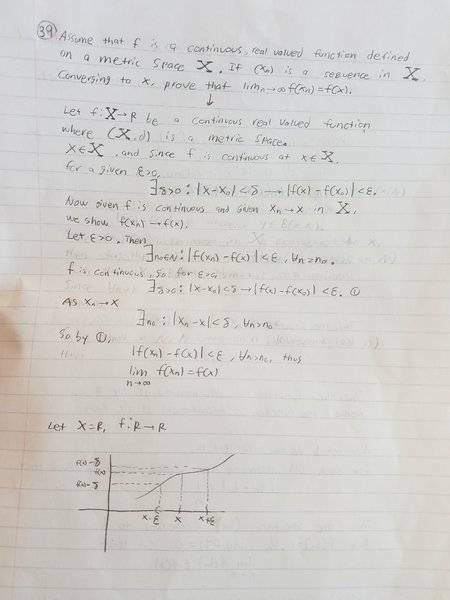# Assume that f is a continuous, real-valued function

Assume that f is a continuous, real-valued function defined on a metric space X. If {xn} is a sequence in X converging to x, prove that Limn→∞f(xn) = f(x).

Here is my attempt, but I am not sure if it is correct.#### Attachments

Svein
The usual way is to use the definitions. What you need to prove is that given any ε>0, there is an N such that $(\forall n>N)(\vert f(x_{n})-f(x)\vert <\epsilon)$. You do this in steps:
• First, since f is continuous, you can find a δ...
• Then since $\lim_{n\rightarrow\infty}x_{n}=x$, there is an N such that...
• Therefore...
Fill in the rest of the arguments!

•member 587159
member 587159
Assume that f is a continuous, real-valued function defined on a metric space X. If {xn} is a sequence in X converging to x, prove that Limn→∞f(xn) = f(x).

Here is my attempt, but I am not sure if it is correct.

View attachment 224230

Anu proof will have to use the definition. Where did you pick an epsilon greater than 0? It is weird to pick ##n##. I suggest you follow the proof strategy suggested by @Svein, and after you are done with the proof, convince yourself that the same proof also applies where the codomain is an arbitrary metric space (i.e. change the absolute values with a distance function and look careful that you never used something else)

Here is my new solution, but I'm not sure if the graph I put is of any use, but if you can, please put up a similar graph to the one I put to help better understand.#### Attachments

member 587159
Here is my new solution, but I'm not sure if the graph I put is of any use, but if you can, please put up a similar graph to the one I put to help better understand.
View attachment 224259

Why ##\exists n_0: |f(x_n)-f(x)| < \epsilon \forall n \geq n_0##? That shouldn't be included in your proof at the beginning, unless you explicitely say that this is what you are going to prove. Also, your lack of the ##\forall x \in X## quantifier is not so good. You should include it. But for the rest, the argument seems fine.